# Kobayashi hyperbolicity

(diff) ← Older revision | Latest revision (diff) | Newer revision → (diff)

domain without large complex discs

Kobayashi hyperbolicity describes in a precise sense whether a complex manifold contains arbitrarily large copies of a one-dimensional complex disc. Extreme examples are the complex disc and the whole complex plane. The former is an example of a Kobayashi-hyperbolic manifold while the latter has arbitrarily large discs in it and is not Kobayashi hyperbolic.

Denote bythe unit disc in the complex plane.

Letbe a complex manifold,a point inand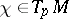a tangent vector. Consider any holomorphic mappingwith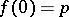,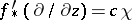. The infinitesimal Kobayashi pseudo-metric is defined by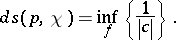The complex manifoldis said to be Kobayashi hyperbolic if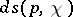is locally bounded below by a strictly positive constant as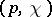varies over the tangent bundle,General references for this area are: [a1], [a2] and [a3].

### Examples.

1) The unit disc. In this case the Kobayashi pseudo-metric coincides with the Poincaré metric (cf. also Poincaré model).

2) More generally than Example 1), any bounded domain inis Kobayashi hyperbolic.

3) At the opposite end, the Kobayashi pseudo-metric of the complex planeas well as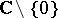vanishes identically.

4) On the other hand,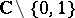is again Kobayashi hyperbolic. The reason for this is that there is a covering ofby the unit disc, and coverings are isometries.

5) The remarkable Brody theorem states that a compact complex manifoldof any dimension is Kobayashi hyperbolic if and only if there is no non-constant holomorphic mapping ofto.

The proof of this theorem starts, assuming non-hyperbolicity, with a sequence of holomorphic mappings of the unit disc towith derivatives atconverging to infinity. Next one does a suitable scaling to normalize to a sequence which has derivative of length one atand which converges to a mapping on the whole plane.

6) Generalizations of 4) and 5) have been obtained by M. Green, see [a2], who gave some criteria ensuring that the complement of a finite family of complex hypersurfaces in complex projective space is Kobayashi hyperbolic.

7) The hyperbolicity ofhas traditionally been a useful tool in complex dynamics in one dimension. Recently, Kobayashi hyperbolicity has been used in complex dynamics in higher dimensions. For example, T. Ueda, see [a3], showed that all Fatou components, i.e. sets of normality of iterates, of a holomorphic mapping on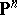are Kobayashi hyperbolic.

How to Cite This Entry:
Kobayashi hyperbolicity. Encyclopedia of Mathematics. URL: http://encyclopediaofmath.org/index.php?title=Kobayashi_hyperbolicity&oldid=16571
This article was adapted from an original article by J.E. FornÃ¦ss (originator), which appeared in Encyclopedia of Mathematics - ISBN 1402006098. See original article